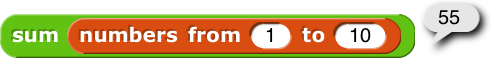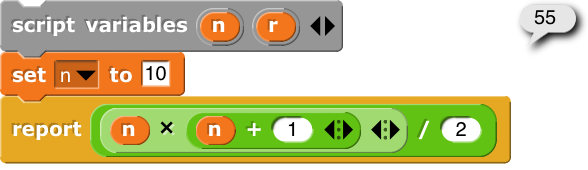# A more effective weighted dice roll

Here’s my issue, I need to make a weighted dice roll that randomly selects an item from a list based on how big it is, my Let’s chance blocks would work really good for this, however they aren’t good for big weights or very large datasets

I think there’s a better way of speeding this up that isn’t the let’s chance blocks

What I’m trying to do is for example if I did “list 1 to 10” the item at the end of the list would have a “10” higher chance than the item at the start (the way the lets chance blocks work is by taking the value and adding that value repeatedly to the list the number of times it’s weighted, then picking a random item)

TLDR: I need a way to pick a random list index weighted at the end of a list

I’m not sure I fully understand what you’re looking for. Can you present an example of the input and what the resulting odds are?

Are you looking for something like … ?

with:

See also here (check posts 15, 17 for faster versions of CUMULATIVE, with many buckets).

Basically all I was trying to do was pick a random list index with the items at the end of the list being more likely to be picked. The lets chance blocks I made do work however they are very slow when working with large datasets due to the way they work, and those blocks are ment for text items not numbers

Do you mean the first item has probability 1 / sum to be picked, 2nd item: 2 / sum, etc., with sum being the sum of 1, 2, 3 … n (= length of list)?

That’s not too difficult.
The sum as a function of the list length = n × (n + 1) / 2.

For example:So you use

to pick a random number.

Next, you need to convert it to the right index.
That’s a matter of solving a quadratic equation: r = n × ( n + 1) / 2,
or its equivalent: n2, + n - 2 r = 0.
Using high-school math, the quadratic formula: n = (√ (1 + 8 r) - 1) / 2.

Example:

So in order to find the right index for any generated random number, use:

I think I came up with another way to do this that fits better with what I need (unfortunately if this is correct I don’t understand it sorryyyy)

I’m curious as to what you invented yourselfThe thing that I was creating worked more effectivy using an entirely different thing not at all related to this than doing this

Too bad ... it was an interesting question with a good solution (even if I say so myself)I think that's much too heavy a weighting. I mean, I don't know what the underlying problem is, but having one value 10 times as likely as another value won't model much in the real world. My first impulse would be to use ceiling(log n) rather than n as the weight for the nth item. That grows as n grows: (log (n+1)) > (log n). But it grows much more slowly than n. If we're taking logs base 10, log(1000)=3, and 3 is much smaller than 1000.

Ai.

Basically the point is that it’s more likely to change/add things near the end of its actions than at the beginning because if it’s equally likely to change things at the beginning of it’s actions it will learn slower, only after it acoomplishes it’s goal will we change it to the next phase

I plan on making a proper ai that’s more than just NEAT and is a version of NEAT that uses a seperate ai/algorithm to weight where the list is being modified by taking the point value at each step and then using the outlier as the weighted point (because that’s the spot with the most decrease in points)

If you mean that your AI should be more likely to apply stuff that it has recently learned, a (negative) exponential probability distribution might be more suitable.

Application example
Suppose the AI stores everything it learns in a list, using IN FRONT OF to add new “learnings”. Them item 1 is the most recent learning, e.g. item 10 is the earliest.

The probability of item (i) to be selected is numerator (i) / Σ (with Σ is the sum of all numerators). Let’s take 1 as numerator for item 1, so the probability of item 1 to be selected is 1 / Σ.

The probability of each further item to be selected is the probability of the previous item multiplied by a constant factor that is generally < 1.

Now take e.g. 0.8 for the factor. Then probability (2) = 0.8 × probabilty (1) = 0.8 / Σ. Probability (3) = 0.8 × probability (2) = 0.64 / Σ […] probability (10) = 0.89 / Σ = 0.13 / Σ (or 0.13 × probabilty (1)). And so on, when more learnings (items) are added to the list.

What it means
With many items, Σ approaches 1 / (1 - 0.8) = 5. So over time the probability of item 1 (the newest learning) to be selected becomes 20%, and everything after item 13 has a probability < 1%. If you would like a less steep descent, try a higher value for the factor, e.g. 0.9, 0.95, or even 0.99 - just don’t cross 1.

Yes, I understand that. The question is how much more likely. Using the index as the weighting, I think, means that it'll essentially never pick the first item in the list.

Let's say there are 10 items in the list. Then the weighted list (the one with duplicate items) has 1+2+···+10 = 55 items, and the probability of picking the first item (which isn't duplicated) is 1/55 = .01818... which is tiny. If you have a really big list, it's even worse.

To make the weighting less extreme, make the number of copies of item n be some function of n that grows more slowly than $$id(n)=n$$, such as $$\sqrt n$$ or, as I suggested, $$\log(n)$$, which is most commonly used in this situation. (Actually $$\log(n)+1$$, because log(1)=0, not 1.) $$\sqrt{10}≈3.16$$, so the weighted list has $$\lceil\sqrt 1\rceil+\lceil\sqrt 2\rceil+\cdots+\lceil\sqrt{10}\rceil=26$$\$items, so the probability of getting the first item is 1/26, and the probability of getting the last item is only four times ($$\lceil\sqrt{10}\rceil$$) the probability of getting the first item.

log(10)+1=2, so if you use log, the last item comes up twice as often as the first item. Oh, oops, because of taking the ceiling, every weighting is either 1 or 2, so actually log only works well on large lists.

Geez, I hope you mean log rather than exp. 210=1024, so the last item would be 1024 times as likely as the first item!

But they actually don't need to calculate probabilities, just to calculate weights, which is simpler, I think.

I was a bit fuzzy in my use of terms like factor and exponent … I do mean negatively exponential, so descending. This is actually how a moving average is often calculated.